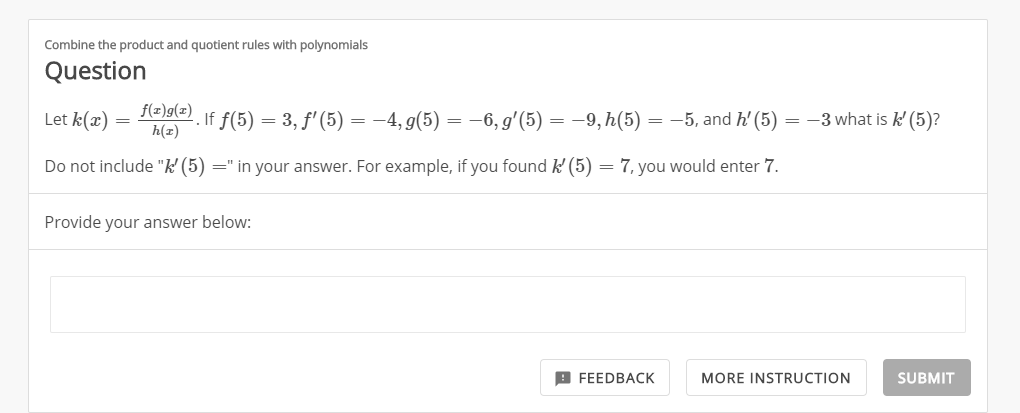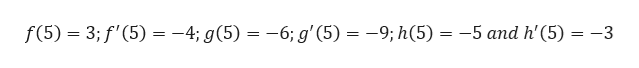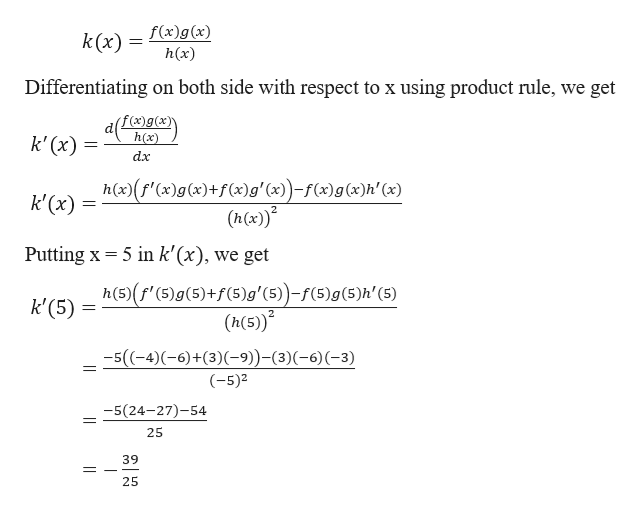Combine the product and quotient rules with polynomialsQuestionf@}g[#) If f(5) = 3, f' (5) = -4, g(5)h(z)=-6, g'(5)-9, h(5)-5, and h' (5) = -3 what is k (5)?Let k(æ)=Do not include "k' (5) =" in your answer. For example, if you found k (5)7, you would enter 7=Provide your answer below:SUBMITFEEDBACKMORE INSTRUCTION

Questionhelp_outlineImage TranscriptioncloseCombine the product and quotient rules with polynomials Question f@}g[#) If f(5) = 3, f' (5) = -4, g(5) h(z) =-6, g'(5)-9, h(5) -5, and h' (5) = -3 what is k (5)? Let k(æ) = Do not include "k' (5) =" in your answer. For example, if you found k (5) 7, you would enter 7 = Provide your answer below: SUBMIT FEEDBACK MORE INSTRUCTION fullscreen
Step 1

Given,help_outlineImage Transcriptionclosef(5) 3;f'(5) -4; g(5) = -6; g'(5) 9; h(5) -5 and h'(5) 3 _ fullscreen
Step 2

Now,

...help_outlineImage Transcriptionclosek(x) f(x)g(x) h(x) Differentiating on both side with respect to x using product rule, we get f(x)g(x)\ h(x) k' (x) dx h(x)(f'(x)g(x)+f(x)g' (x)-f(x)g(x)h' (x) (h(x)* k'(x) 5 in k'(x), we get Putting x h(5)(f' (5)g(5)+f(5)g' (5))-f(5)g (5)h'(5) (h(5) k'(5) 5((-4)(-6)+(3)(-9)-(3)(-6)(-3) (-5)2 -5(24-27)-54 25 39 25 fullscreen

Want to see the full answer?

See Solution

Want to see this answer and more?

Our solutions are written by experts, many with advanced degrees, and available 24/7

See Solution
Tagged in

Derivative